This site uses cookies. By continuing to browse the ConceptDraw site you are agreeing to our Use of Site Cookies.
ConceptDraw Samples | Software Development — Object-Role Modeling (ORM) Diagrams

# Object-Role Modeling (ORM) Diagrams

The Object-Role Modeling (ORM) diagrams are created using ConceptDraw DIAGRAM diagramming and vector drawing software enhanced with Object-Role Modeling (ORM) Diagrams solution from ConceptDraw Solution Park.

ConceptDraw DIAGRAM provides export of vector graphic multipage documents into multiple file formats: vector graphics (SVG, EMF, EPS), bitmap graphics (PNG, JPEG, GIF, BMP, TIFF), web documents (HTML, PDF), PowerPoint presentations (PPT), Adobe Flash (SWF).

## Tutorials and Solutions:

Video Tutorials: ConceptDraw Solution Park

## Sample 1: Constituent Geometry ORM Diagram

Object-Role Modeling (ORM) Diagrams sample: Constituent Geometry ORM Diagram.

This example is created using ConceptDraw DIAGRAM diagramming software enhanced with Object-Role Modeling (ORM) Diagrams solution from ConceptDraw Solution Park.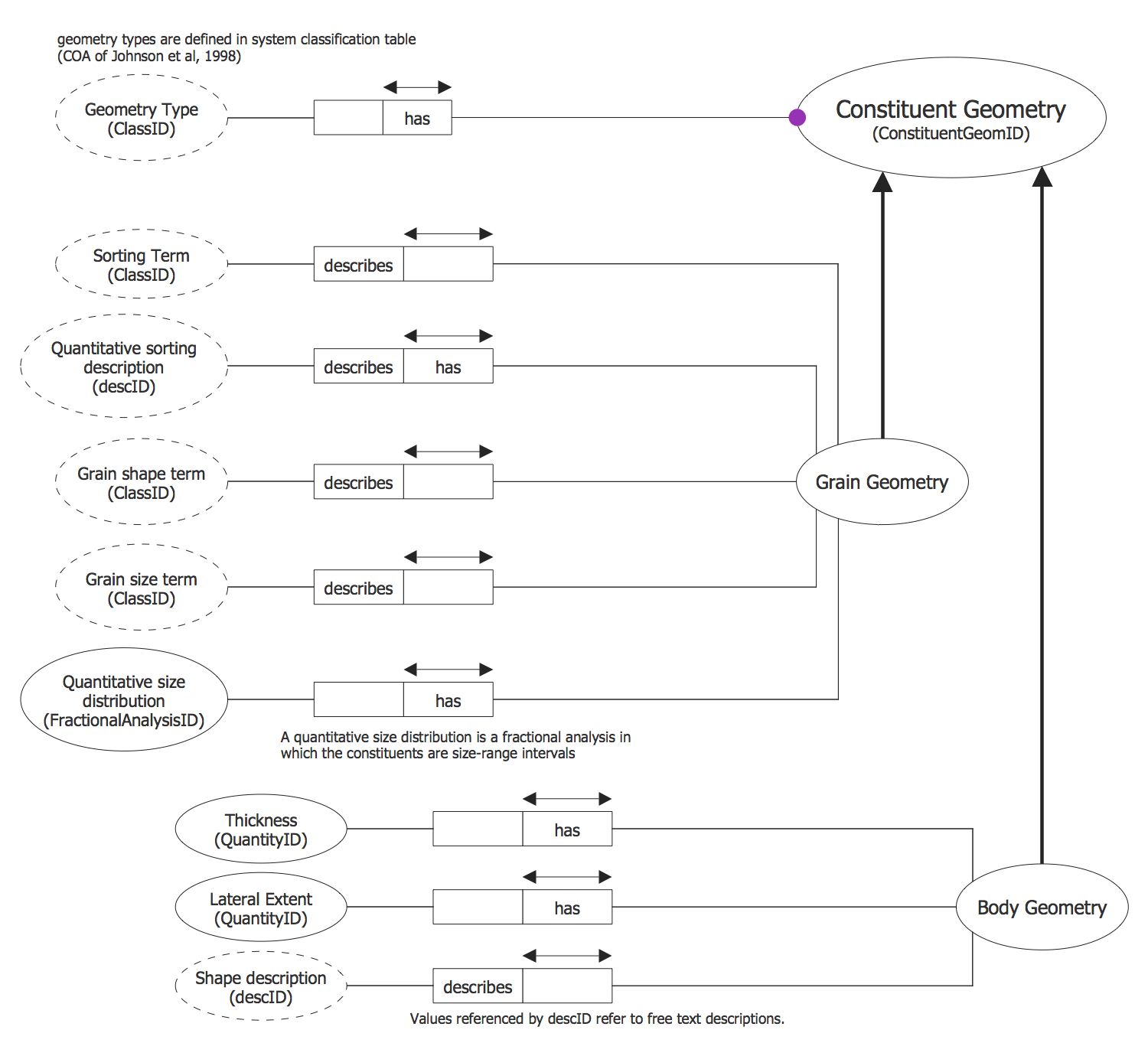## Sample 2: Disjunctive Mandatory Limit ORM Diagram

Object-Role Modeling (ORM) Diagrams sample: Disjunctive Mandatory Limit ORM Diagram.

This example is created using ConceptDraw DIAGRAM diagramming software enhanced with Object-Role Modeling (ORM) Diagrams solution from ConceptDraw Solution Park.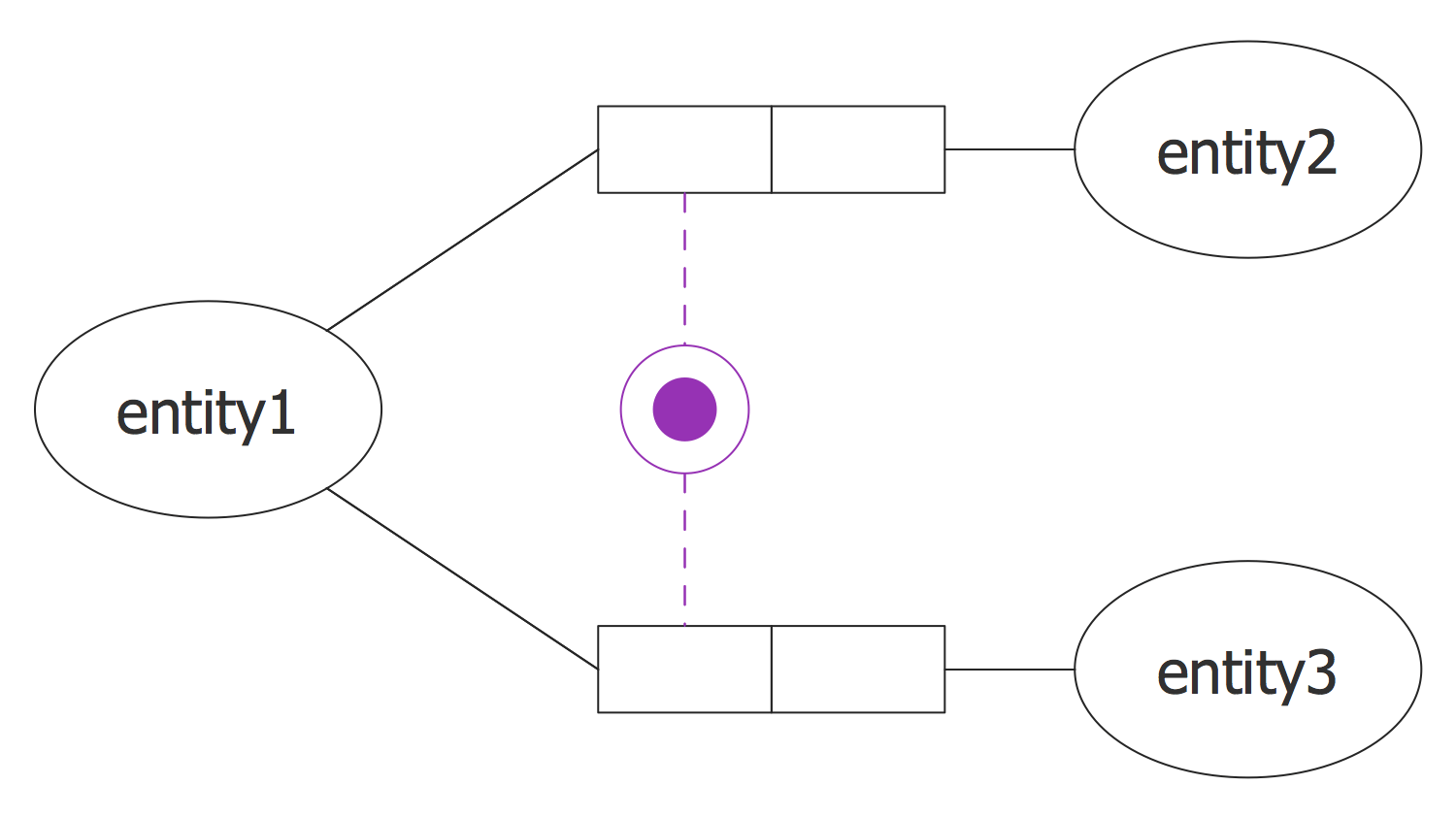## Sample 3: Employee ORM Diagram

Object-Role Modeling (ORM) Diagrams sample: Employee ORM Diagram.

This example is created using ConceptDraw DIAGRAM diagramming software enhanced with Object-Role Modeling (ORM) Diagrams solution from ConceptDraw Solution Park.## Sample 4: Fractional Analysis ORM Diagram

Object-Role Modeling (ORM) Diagrams sample: Fractional Analysis ORM Diagram.

This example is created using ConceptDraw DIAGRAM diagramming software enhanced with Object-Role Modeling (ORM) Diagrams solution from ConceptDraw Solution Park.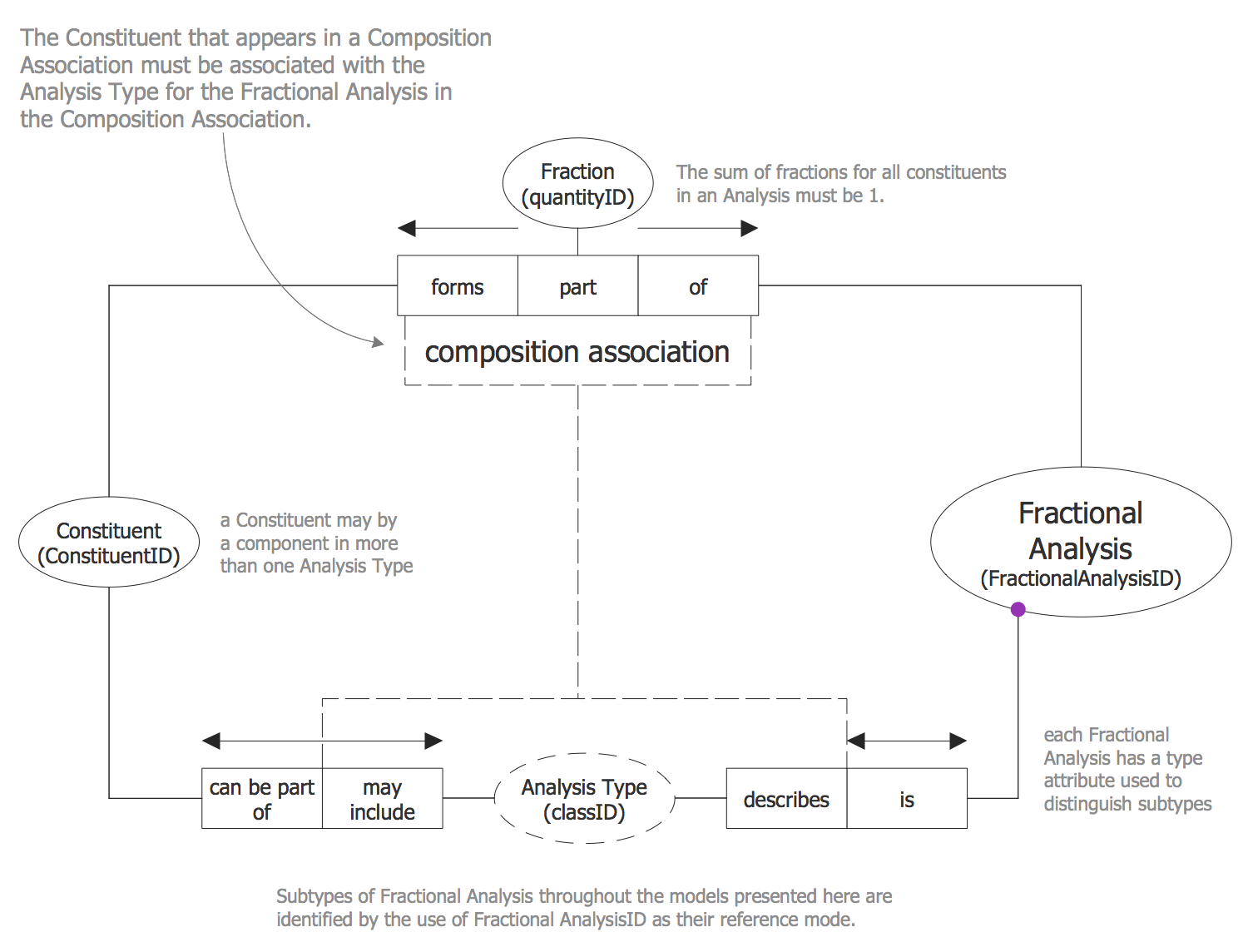## Sample 5: General Lithology ORM Diagram

Object-Role Modeling (ORM) Diagrams sample: General Lithology ORM Diagram.

This example is created using ConceptDraw DIAGRAM diagramming software enhanced with Object-Role Modeling (ORM) Diagrams solution from ConceptDraw Solution Park.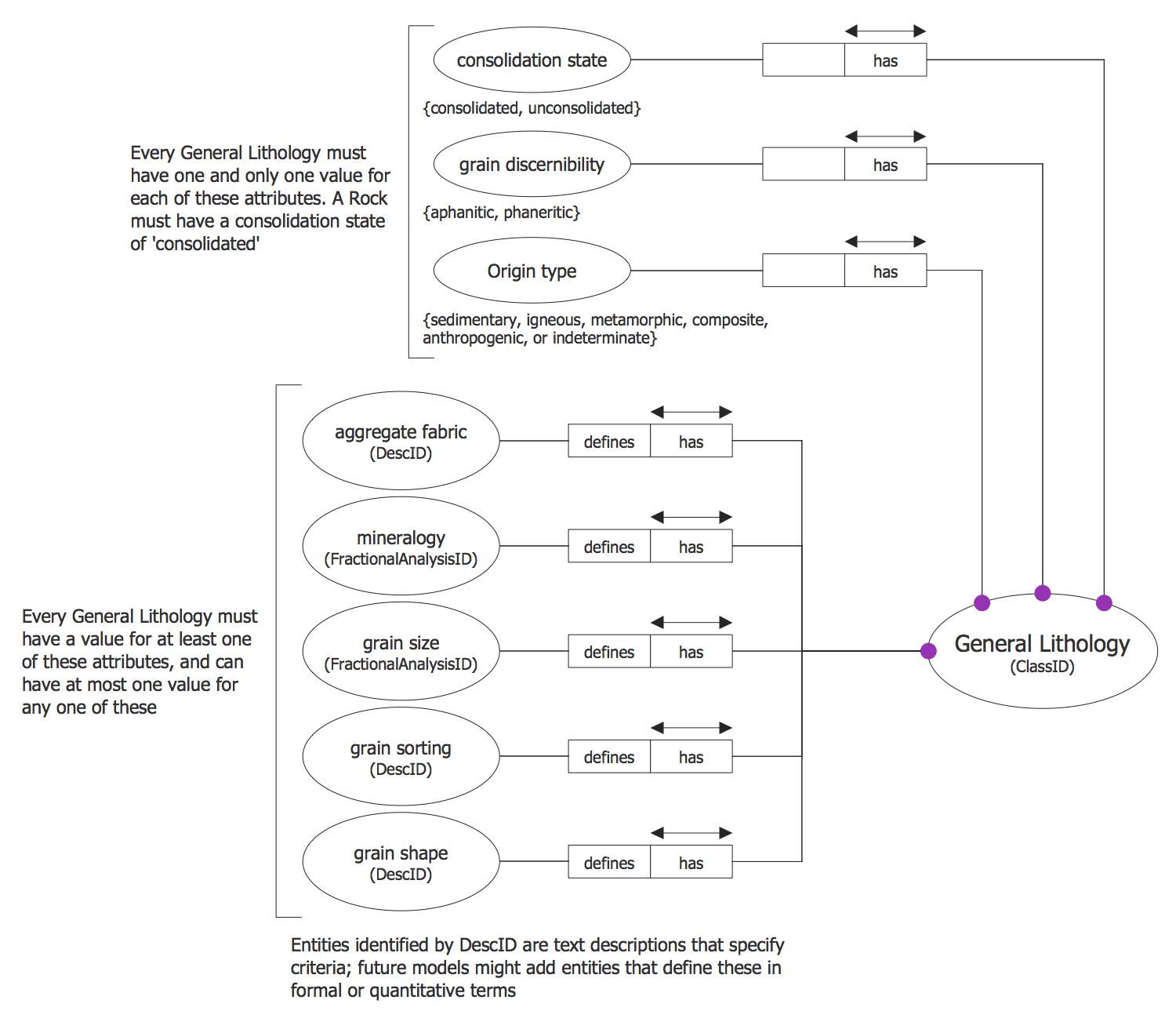## Sample 6: Geologic Surface ORM Diagram

Object-Role Modeling (ORM) Diagrams sample: Geologic Surface ORM Diagram.

This example is created using ConceptDraw DIAGRAM diagramming software enhanced with Object-Role Modeling (ORM) Diagrams solution from ConceptDraw Solution Park.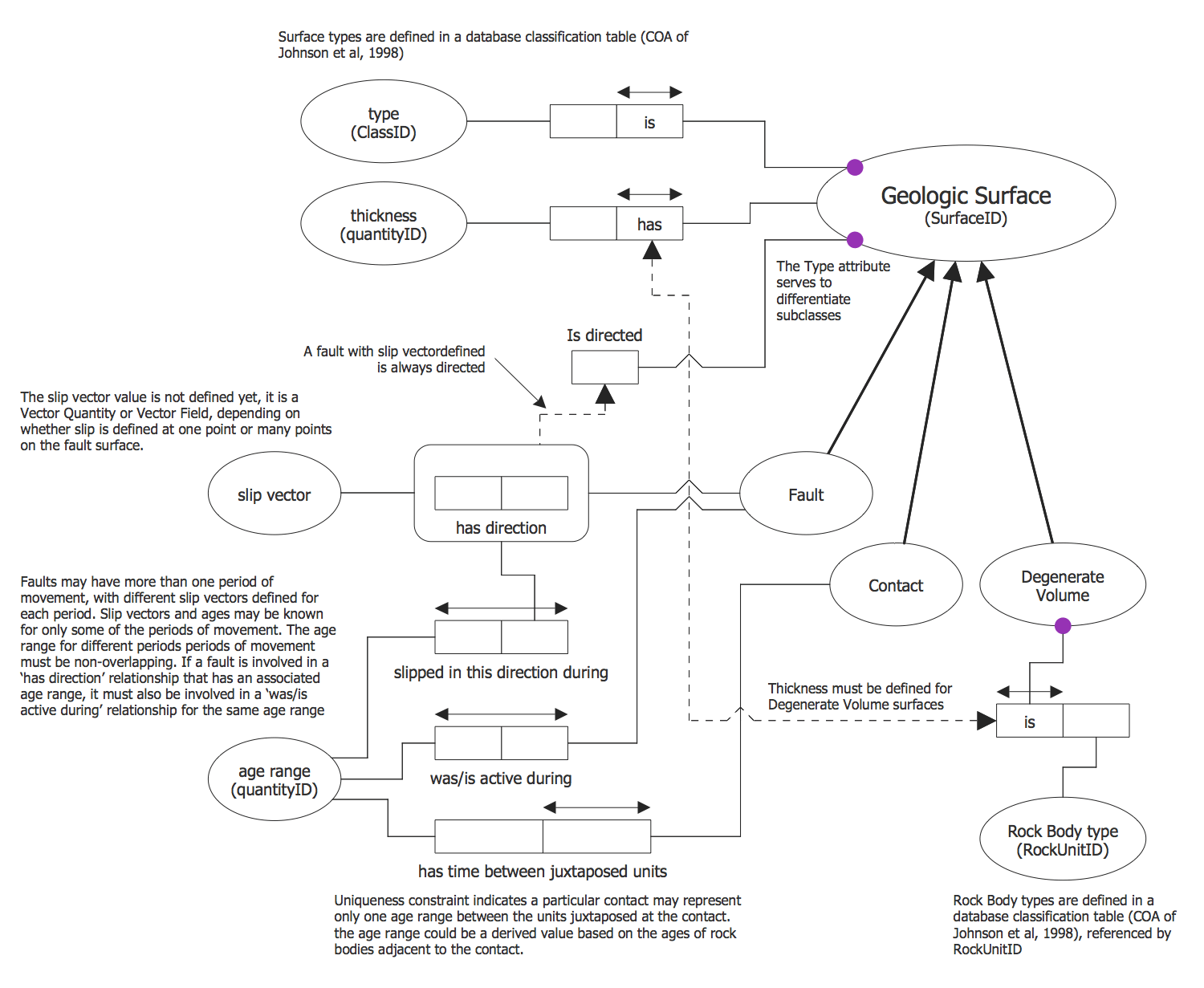## Sample 7: Object-Role Modeling Example

Object-Role Modeling (ORM) Diagrams sample: Object-Role Modeling Example.

This example is created using ConceptDraw DIAGRAM diagramming software enhanced with Object-Role Modeling (ORM) Diagrams solution from ConceptDraw Solution Park.## Sample 8: Object-Role Model Overview

Object-Role Modeling (ORM) Diagrams sample: Object-Role Model Overview.

This example is created using ConceptDraw DIAGRAM diagramming software enhanced with Object-Role Modeling (ORM) Diagrams solution from ConceptDraw Solution Park.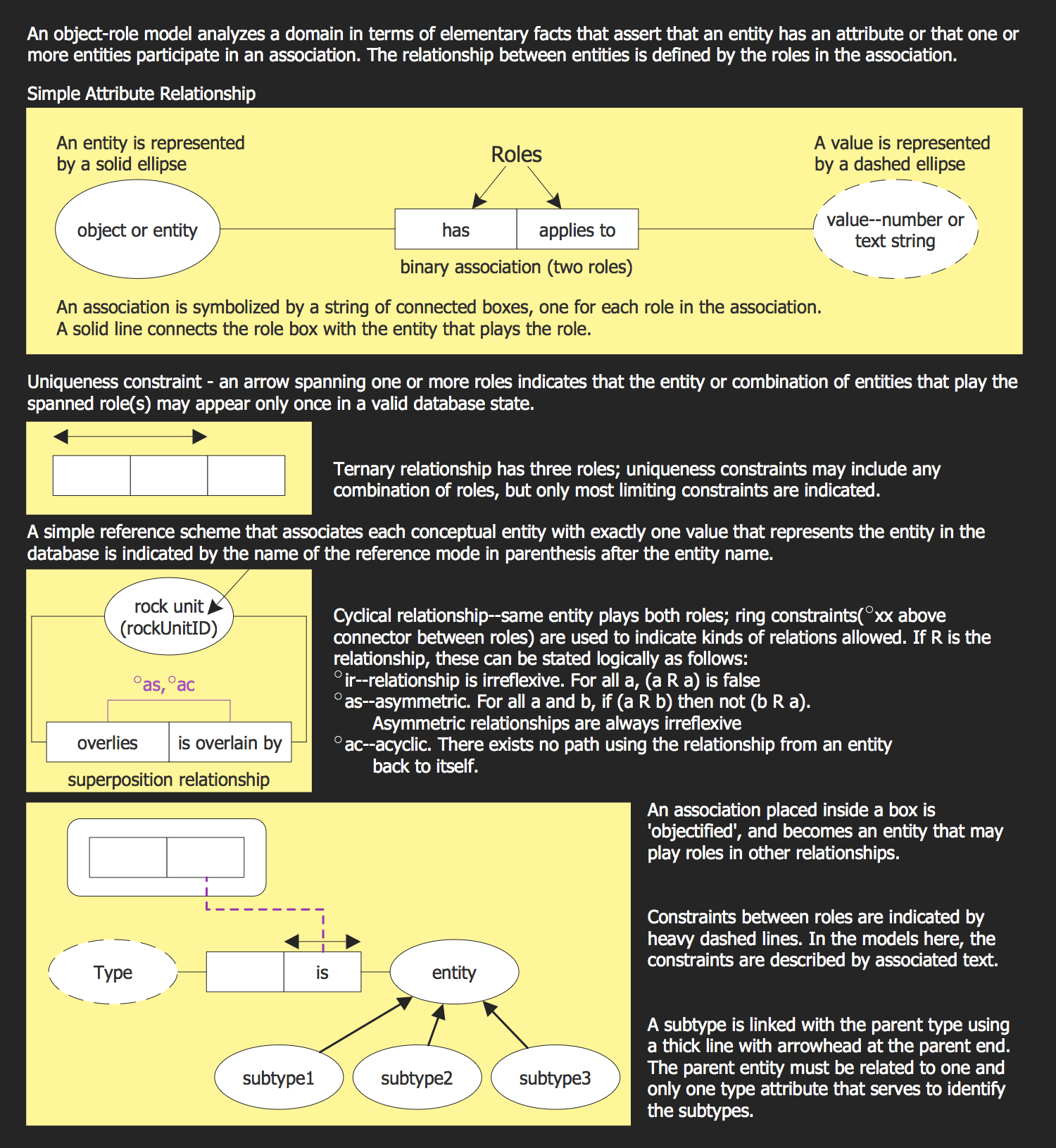## Sample 9: Particular Lithology ORM Diagram

Object-Role Modeling (ORM) Diagrams sample: Particular Lithology ORM Diagram.

This example is created using ConceptDraw DIAGRAM diagramming software enhanced with Object-Role Modeling (ORM) Diagrams solution from ConceptDraw Solution Park.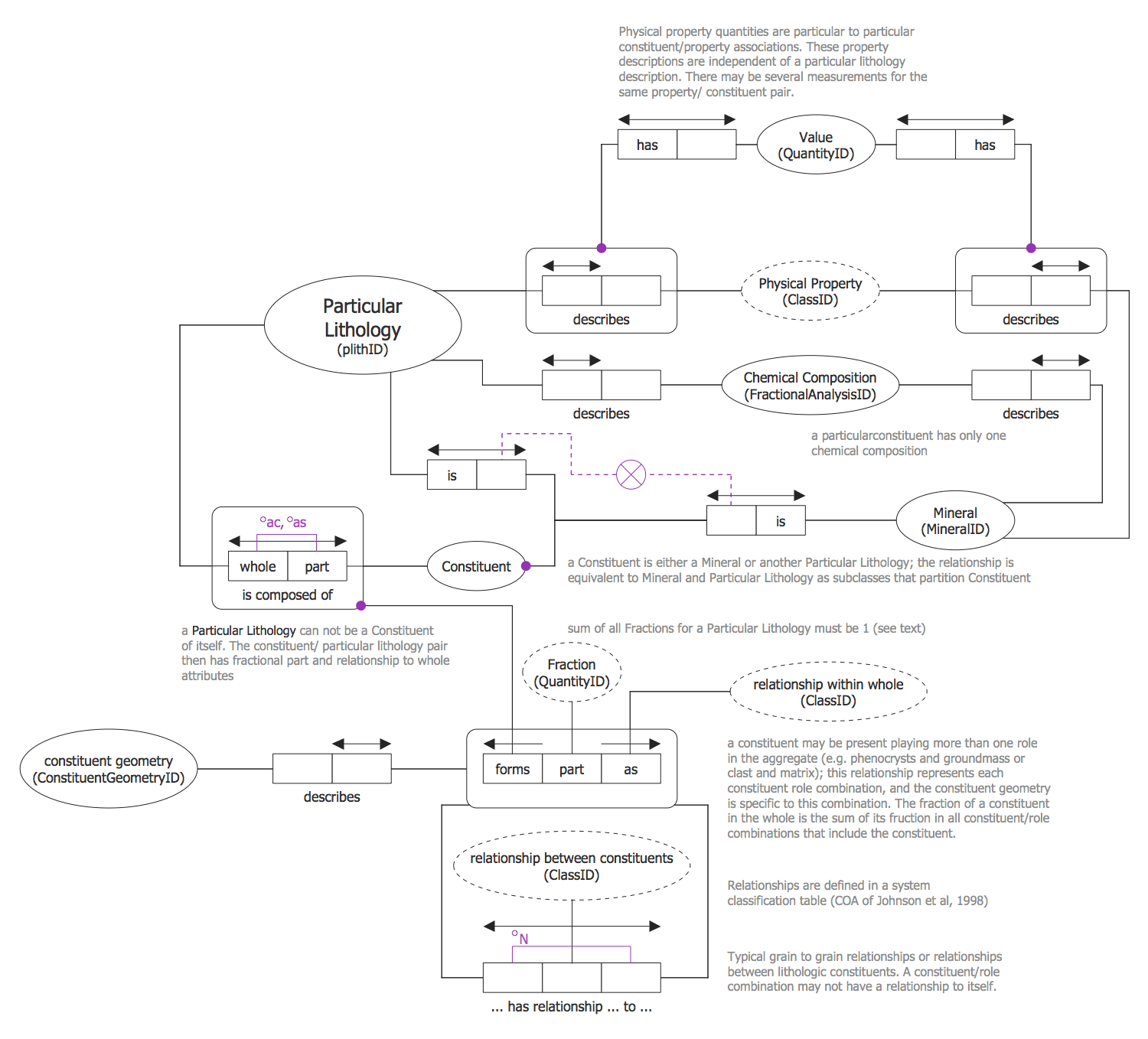## Sample 10: Scalar Quantity ORM Diagram

Object-Role Modeling (ORM) Diagrams sample: Scalar Quantity ORM Diagram.

This example is created using ConceptDraw DIAGRAM diagramming software enhanced with Object-Role Modeling (ORM) Diagrams solution from ConceptDraw Solution Park.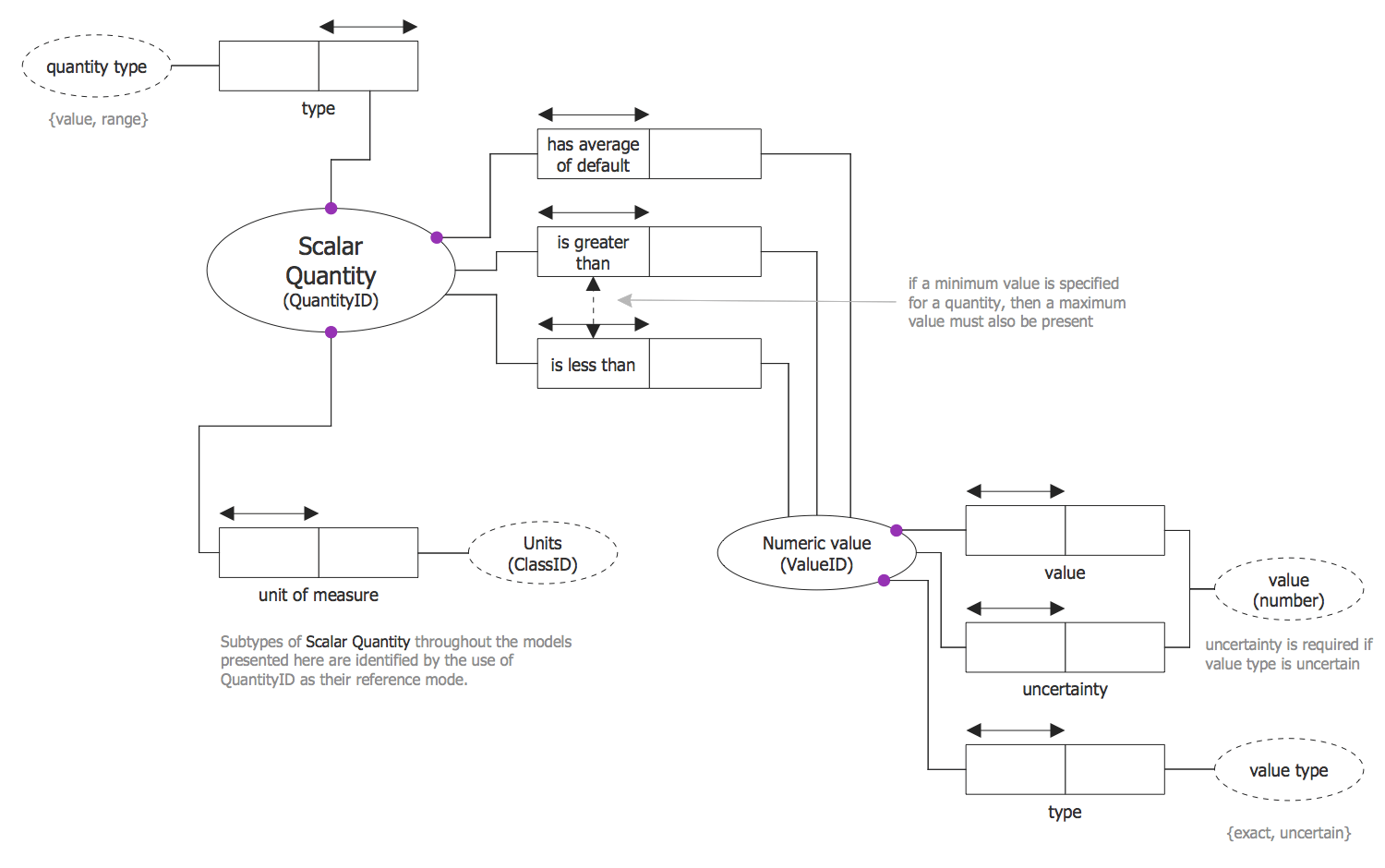All samples are copyrighted CS Odessa's.

Usage of them is covered by Creative Commons “Attribution Non-Commercial No Derivatives” License.

The text you can find at: https://creativecommons.org/licenses/by-nc-nd/3.0